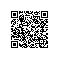# SEO标准模板

SEO标准模板

<html>
<meta http-equiv="Content-Type" content="text/html; charset=gb2312">
<title>$$网页内容标题$$——$$频道标题$$——$$网站名$$</title>
<meta name="Keywords" content="$$网页内容标题$$ $$频道标题$$">
<meta name="Description" content="描述性语句，$$网页内容标题$$ 描述性语句">
<body>
$$网站导航$$

<h1>$$网页内容标题$$</h1>

$$网页内容$$

<br>
$$相关内容链接$$

<br>

$$值得全站网民方便查看的固定内容链接$$

<br>
$$隐私条款，友情链接，版权声明，http://www.wangjianran.com/ 联系方式，留言等等$$
</body>
</html>

seo标准模板 注释：
1 css样式表代码尽量和htm、asp等文件分离
2 js代码也尽量和htm、asp等文件分离
3 模块化，规范化。世界上没有高深的知识，全是基础知识。基本而重要的html代码知识http://tech.china.com/zh_cn/netschool/homepage/html/599/20010205/html01.html
4 每个标记都应该出现在应该出现的位置。
5 还可以给不同的内容块加大div包围标记，运用css控制内容布局。这样可以较少网页大小，方便搜索引擎索引。使用钉钉扫一扫加入圈子
+ 订阅